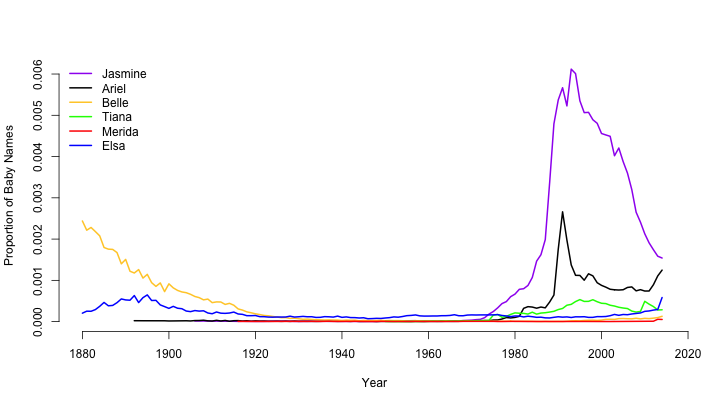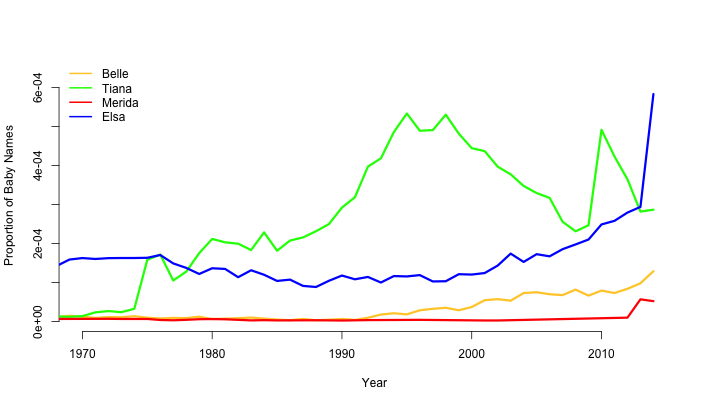# Popularity of the Names of Disney Princesses

This is a small exploration of the babynames package which contains a dataset tracking how many children were born with a certain name according to the US Social Security Administration. I thought it would be interesting to look at the popularity of the names of the Disney Princesses. Spikes in popularity roughly correspond to when their respective movies were released.

library(dplyr)
library(babynames)

# I can show you the world...

jasmine <- babynames %>%
filter(name == "Jasmine", sex == "F")

ariel <- babynames %>%
filter(name == "Ariel", sex == "F")

belle <- babynames %>%
filter(name == "Belle", sex == "F")

tiana <- babynames %>%
filter(name == "Tiana", sex == "F")

merida <- babynames %>%
filter(name == "Merida", sex == "F")

elsa <- babynames %>%
filter(name == "Elsa", sex == "F")

plot(jasmine$year, jasmine$prop, type = "l", lwd = 2,
col = "Purple", xlim = c(1880, 2015), xlab = "Year",
ylab = "Proportion of Baby Names", bty = "n")

lines(ariel$year, ariel$prop, type = "l", lwd = 2,
xlab = "Year", ylab = "Proportion of Baby Names")

lines(belle$year, belle$prop, type = "l", lwd = 2,
col = "#FFCC33")

lines(tiana$year, tiana$prop, type = "l", lwd = 2,
col = "green")

lines(merida$year, merida$prop, type = "l", lwd = 2,
col = "red")

lines(elsa$year, elsa$prop, type = "l", lwd = 2,
col = "blue")

legend("topleft", legend = c("Jasmine", "Ariel", "Belle",
"Tiana", "Merida", "Elsa"),
lwd = rep(2, 6), col = c("Purple", "Black", "#FFCC33",
"Green", "Red", "Blue"),
bty = "n")# Shining, shimmering, splendid

plot(belle$year, belle$prop, type = "l", lwd = 3,
col = "#FFCC33", xlim = c(1970, 2015),
ylim = c(0, max(elsa$prop)), bty = "n", xlab = "Year", ylab = "Proportion of Baby Names") lines(tiana$year, tiana$prop, type = "l", lwd = 3, col = "green") lines(merida$year, merida$prop, type = "l", lwd = 3, col = "red") lines(elsa$year, elsa\$prop, type = "l", lwd = 3,
col = "blue")

legend("topleft", legend = c("Belle",
"Tiana", "Merida", "Elsa"),
lwd = rep(2, 4), col = c("#FFCC33",
"Green", "Red", "Blue"),
bty = "n")# Tell me data scientist, now when did you last let your model decide?